# 7.4 The other trigonometric functions  (Page 8/14)

 Page 8 / 14

Find the area of the sector of a circle with diameter 32 feet and an angle of $\text{\hspace{0.17em}}\frac{3\pi }{5}\text{\hspace{0.17em}}$ radians.

For the following exercises, find the angle between $\text{\hspace{0.17em}}0°\text{\hspace{0.17em}}$ and $\text{\hspace{0.17em}}\text{360°}\text{\hspace{0.17em}}$ that is coterminal with the given angle.

$420°$

$60°$

$-80°$

For the following exercises, find the angle between 0 and $\text{\hspace{0.17em}}2\pi \text{\hspace{0.17em}}$ in radians that is coterminal with the given angle.

$-\text{\hspace{0.17em}}\frac{20\pi }{11}$

$\frac{2\pi }{11}$

$\frac{14\pi }{5}$

For the following exercises, draw the angle provided in standard position on the Cartesian plane.

$-210°$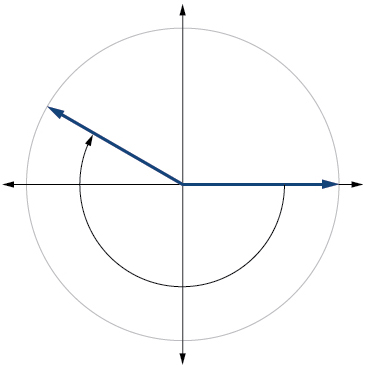$75°$

$\frac{5\pi }{4}$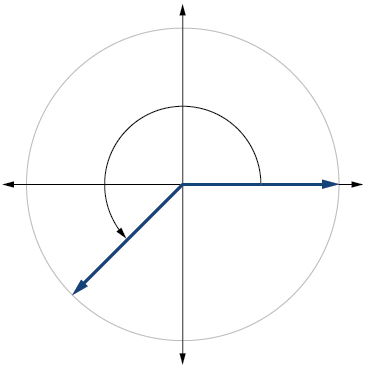$-\frac{\pi }{3}$

Find the linear speed of a point on the equator of the earth if the earth has a radius of 3,960 miles and the earth rotates on its axis every 24 hours. Express answer in miles per hour. Round to the nearest hundredth.

1036.73 miles per hour

A car wheel with a diameter of 18 inches spins at the rate of 10 revolutions per second. What is the car's speed in miles per hour? Round to the nearest hundredth.

## Right Triangle Trigonometry

For the following exercises, use side lengths to evaluate.

$\mathrm{cos}\text{\hspace{0.17em}}\frac{\pi }{4}$

$\frac{\sqrt{2}}{2}$

$\mathrm{cot}\text{\hspace{0.17em}}\frac{\pi }{3}$

$\mathrm{tan}\text{\hspace{0.17em}}\frac{\pi }{6}$

$\frac{\sqrt{3}}{3}$

$\mathrm{cos}\left(\frac{\pi }{2}\right)=\mathrm{sin}\left(___°\right)$

$\mathrm{csc}\left(18°\right)=\mathrm{sec}\left(___°\right)$

$72°$

For the following exercises, use the given information to find the lengths of the other two sides of the right triangle.

$\mathrm{cos}\text{\hspace{0.17em}}B=\frac{3}{5},a=6$

$\mathrm{tan}\text{\hspace{0.17em}}A=\frac{5}{9},b=6$

$a=\frac{10}{3},c=\frac{2\sqrt{106}}{3}$

For the following exercises, use [link] to evaluate each trigonometric function.

$\mathrm{tan}\text{\hspace{0.17em}}B$

$\frac{6}{11}$

For the following exercises, solve for the unknown sides of the given triangle.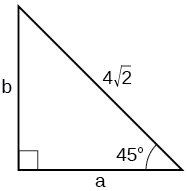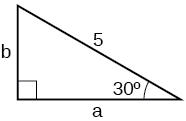$a=\frac{5\sqrt{3}}{2},b=\frac{5}{2}$

A 15-ft ladder leans against a building so that the angle between the ground and the ladder is $\text{\hspace{0.17em}}70°.\text{\hspace{0.17em}}$ How high does the ladder reach up the side of the building? Find the answer to four decimal places.

The angle of elevation to the top of a building in Baltimore is found to be 4 degrees from the ground at a distance of 1 mile from the base of the building. Using this information, find the height of the building. Find the answer to four decimal places.

369.2136 ft

## Unit Circle

Find the exact value of $\text{\hspace{0.17em}}\mathrm{sin}\text{\hspace{0.17em}}\frac{\pi }{3}.$

Find the exact value of $\text{\hspace{0.17em}}\mathrm{cos}\text{\hspace{0.17em}}\frac{\pi }{4}.$

$\frac{\sqrt{2}}{2}$

Find the exact value of $\text{\hspace{0.17em}}\mathrm{cos}\text{\hspace{0.17em}}\pi .$

State the reference angle for $\text{\hspace{0.17em}}300°.\text{\hspace{0.17em}}$

$60°$

State the reference angle for $\text{\hspace{0.17em}}\frac{3\pi }{4}.$

Compute cosine of $\text{\hspace{0.17em}}330°.$

$\frac{\sqrt{3}}{2}$

Compute sine of $\text{\hspace{0.17em}}\frac{5\pi }{4}.$

State the domain of the sine and cosine functions.

all real numbers

State the range of the sine and cosine functions.

## The Other Trigonometric Functions

For the following exercises, find the exact value of the given expression.

$\mathrm{cos}\text{\hspace{0.17em}}\frac{\pi }{6}$

$\frac{\sqrt{3}}{2}$

$\mathrm{tan}\text{\hspace{0.17em}}\frac{\pi }{4}$

$\mathrm{csc}\text{\hspace{0.17em}}\frac{\pi }{3}$

$\frac{2\sqrt{3}}{3}$

$\mathrm{sec}\text{\hspace{0.17em}}\frac{\pi }{4}$

For the following exercises, use reference angles to evaluate the given expression.

$\mathrm{sec}\text{\hspace{0.17em}}\frac{11\pi }{3}$

2

$\mathrm{sec}\text{\hspace{0.17em}}315°$

If $\text{\hspace{0.17em}}\mathrm{sec}\left(t\right)=-2.5,$ what is the $\text{\hspace{0.17em}}\text{sec}\left(-t\right)?$

–2.5

If $\text{\hspace{0.17em}}\text{tan}\left(t\right)=-0.6,$ what is the $\text{\hspace{0.17em}}\text{tan}\left(-t\right)?$

If $\text{\hspace{0.17em}}\text{tan}\left(t\right)=\frac{1}{3},$ find $\text{\hspace{0.17em}}\text{tan}\left(t-\pi \right).$

$\frac{1}{3}$

If $\text{\hspace{0.17em}}\text{cos}\left(t\right)=\frac{\sqrt{2}}{2},$ find $\text{\hspace{0.17em}}\text{sin}\left(t+2\pi \right).$

Which trigonometric functions are even?

cosine, secant

Which trigonometric functions are odd?

## Chapter practice test

Convert $\text{\hspace{0.17em}}\frac{5\pi }{6}\text{\hspace{0.17em}}$ radians to degrees.

$150°$

Convert $\text{\hspace{0.17em}}-620°\text{\hspace{0.17em}}$ to radians.

Find the length of a circular arc with a radius 12 centimeters subtended by the central angle of $\text{\hspace{0.17em}}30°.$

6.283 centimeters

Find the area of the sector with radius of 8 feet and an angle of $\text{\hspace{0.17em}}\frac{5\pi }{4}\text{\hspace{0.17em}}$ radians.

Find the angle between $\text{\hspace{0.17em}}0°\text{\hspace{0.17em}}$ and $\text{\hspace{0.17em}}\text{360°}\text{\hspace{0.17em}}$ that is coterminal with $\text{\hspace{0.17em}}375°.$

$15°$

Find the angle between 0 and $\text{\hspace{0.17em}}2\pi \text{\hspace{0.17em}}$ in radians that is coterminal with $\text{\hspace{0.17em}}-\frac{4\pi }{7}.$

Draw the angle $\text{\hspace{0.17em}}315°\text{\hspace{0.17em}}$ in standard position on the Cartesian plane.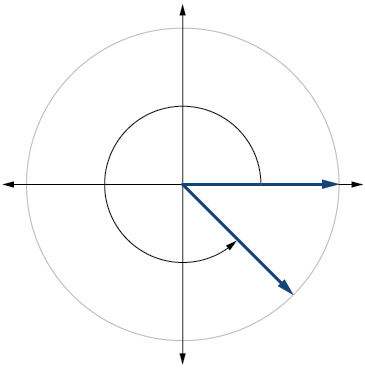Draw the angle $\text{\hspace{0.17em}}-\frac{\pi }{6}\text{\hspace{0.17em}}$ in standard position on the Cartesian plane.

A carnival has a Ferris wheel with a diameter of 80 feet. The time for the Ferris wheel to make one revolution is 75 seconds. What is the linear speed in feet per second of a point on the Ferris wheel? What is the angular speed in radians per second?

3.351 feet per second, $\text{\hspace{0.17em}}\frac{2\pi }{75}\text{\hspace{0.17em}}$ radians per second

Find the missing sides of the triangle $\text{\hspace{0.17em}}ABC:\mathrm{sin}\text{\hspace{0.17em}}B=\frac{3}{4},c=12.$

Find the missing sides of the triangle.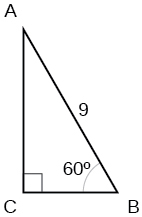$a=\frac{9}{2},b=\frac{9\sqrt{3}}{2}$

The angle of elevation to the top of a building in Chicago is found to be 9 degrees from the ground at a distance of 2000 feet from the base of the building. Using this information, find the height of the building.

Find the exact value of $\text{\hspace{0.17em}}\mathrm{sin}\text{\hspace{0.17em}}\frac{\pi }{6}.$

$\frac{1}{2}$

Compute sine of $\text{\hspace{0.17em}}240°.$

State the domain of the sine and cosine functions.

real numbers

State the range of the sine and cosine functions.

Find the exact value of $\text{\hspace{0.17em}}\mathrm{cot}\text{\hspace{0.17em}}\frac{\pi }{4}.$

1

Find the exact value of $\text{\hspace{0.17em}}\mathrm{tan}\text{\hspace{0.17em}}\frac{\pi }{3}.$

Use reference angles to evaluate $\text{\hspace{0.17em}}\mathrm{csc}\text{\hspace{0.17em}}\frac{7\pi }{4}.$

$\text{\hspace{0.17em}}-\sqrt{2}$

Use reference angles to evaluate $\text{\hspace{0.17em}}\mathrm{tan}\text{\hspace{0.17em}}210°.$

If $\text{\hspace{0.17em}}\text{csc}\text{\hspace{0.17em}}t=0.68,$ what is the $\text{\hspace{0.17em}}\text{csc}\left(-t\right)?$

–0.68

If $\text{\hspace{0.17em}}\text{cos}\text{\hspace{0.17em}}t=\frac{\sqrt{3}}{2},$ find $\text{\hspace{0.17em}}\text{cos}\left(t-2\pi \right).$

Find the missing angle: $\text{\hspace{0.17em}}\mathrm{cos}\left(\frac{\pi }{6}\right)=\mathrm{sin}\left(___\right)$

$\frac{\pi }{3}$

#### Questions & Answers

write down the polynomial function with root 1/3,2,-3 with solution
if A and B are subspaces of V prove that (A+B)/B=A/(A-B)
write down the value of each of the following in surd form a)cos(-65°) b)sin(-180°)c)tan(225°)d)tan(135°)
Prove that (sinA/1-cosA - 1-cosA/sinA) (cosA/1-sinA - 1-sinA/cosA) = 4
what is the answer to dividing negative index
In a triangle ABC prove that. (b+c)cosA+(c+a)cosB+(a+b)cisC=a+b+c.
give me the waec 2019 questions
the polar co-ordinate of the point (-1, -1)
prove the identites sin x ( 1+ tan x )+ cos x ( 1+ cot x )= sec x + cosec x
tanh`(x-iy) =A+iB, find A and B
B=Ai-itan(hx-hiy)
Rukmini
what is the addition of 101011 with 101010
If those numbers are binary, it's 1010101. If they are base 10, it's 202021.
Jack
extra power 4 minus 5 x cube + 7 x square minus 5 x + 1 equal to zero
the gradient function of a curve is 2x+4 and the curve passes through point (1,4) find the equation of the curve
1+cos²A/cos²A=2cosec²A-1
test for convergence the series 1+x/2+2!/9x3ByByByByBy